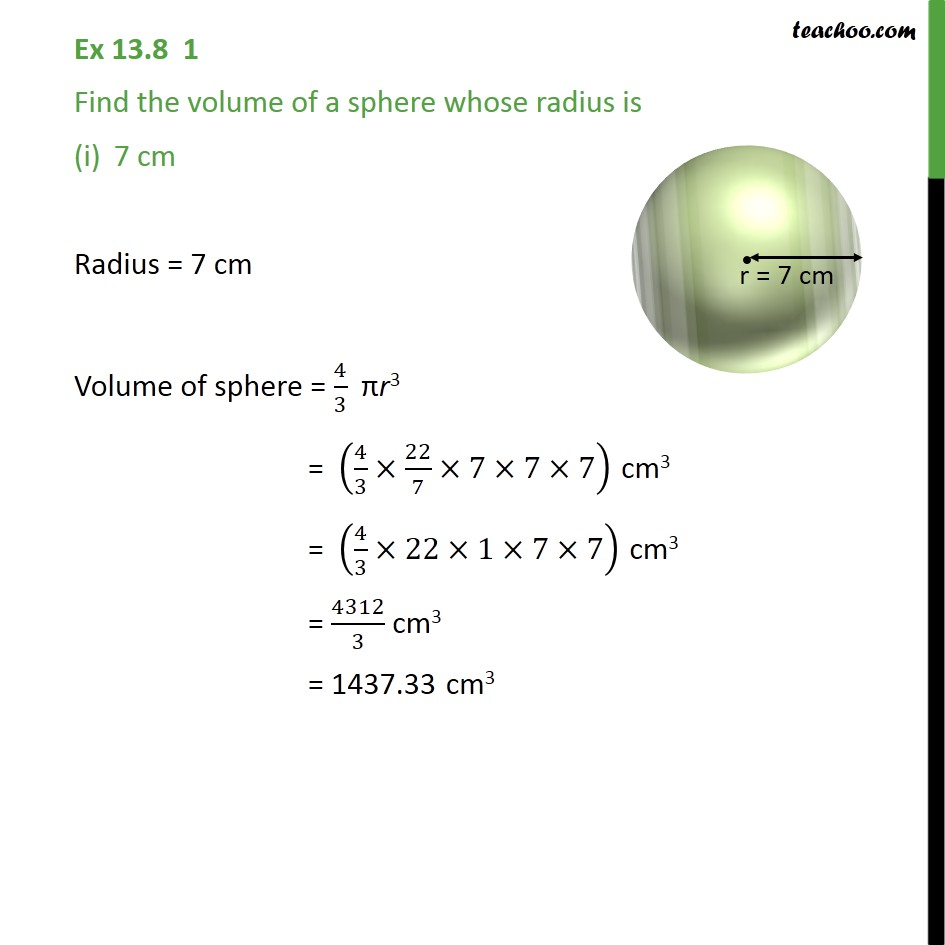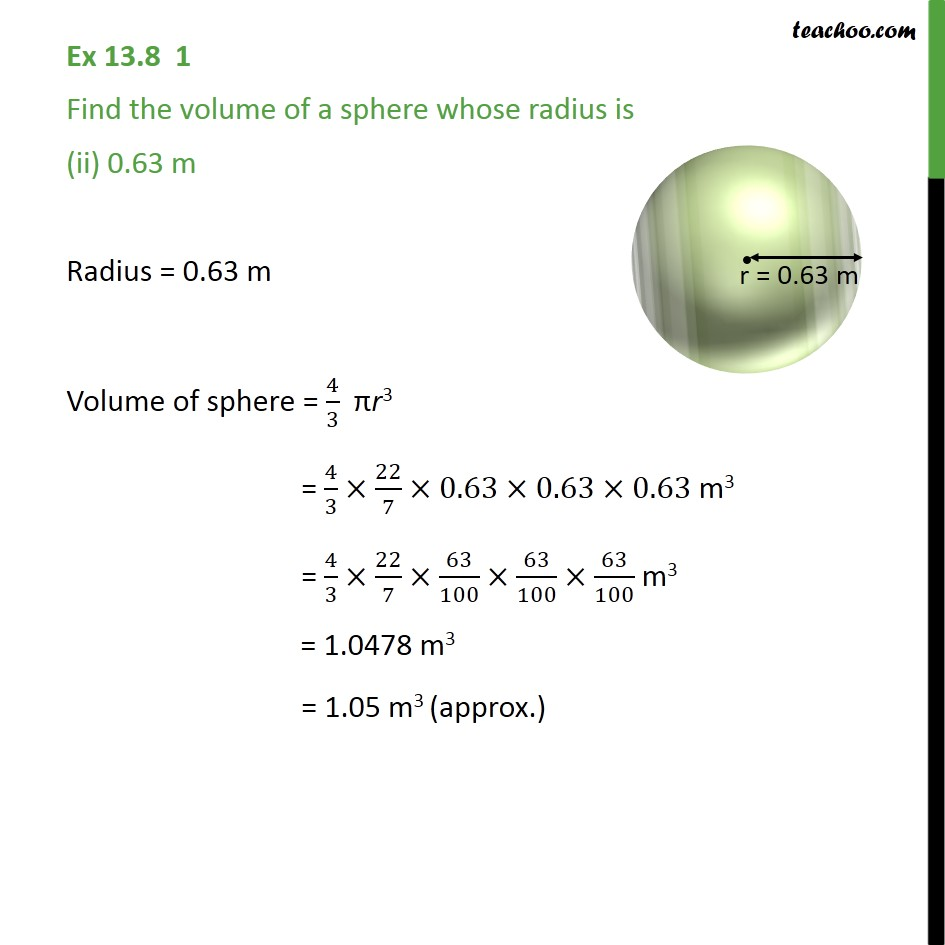Subscribe to our Youtube Channel - https://you.tube/teachoo

1. Chapter 13 Class 9 Surface Areas and Volumes
2. Serial order wise
3. Ex 13.8

Transcript

Ex 13.8 1 Find the volume of a sphere whose radius is 7 cm Radius = 7 cm Volume of sphere = 4/3 r3 = (4/3 22/7 7 7 7) cm3 = (4/3 22 1 7 7) cm3 = 4312/3 cm3 = 1437.33 cm3 Ex 13.8 1 Find the volume of a sphere whose radius is (ii) 0.63 m Radius = 0.63 m Volume of sphere = 4/3 r3 = 4/3 22/7 0.63 0.63 0.63 m3 = 4/3 22/7 63/100 63/100 63/100 m3 = 1.0478 m3 = 1.05 m3 (approx.)

Ex 13.8